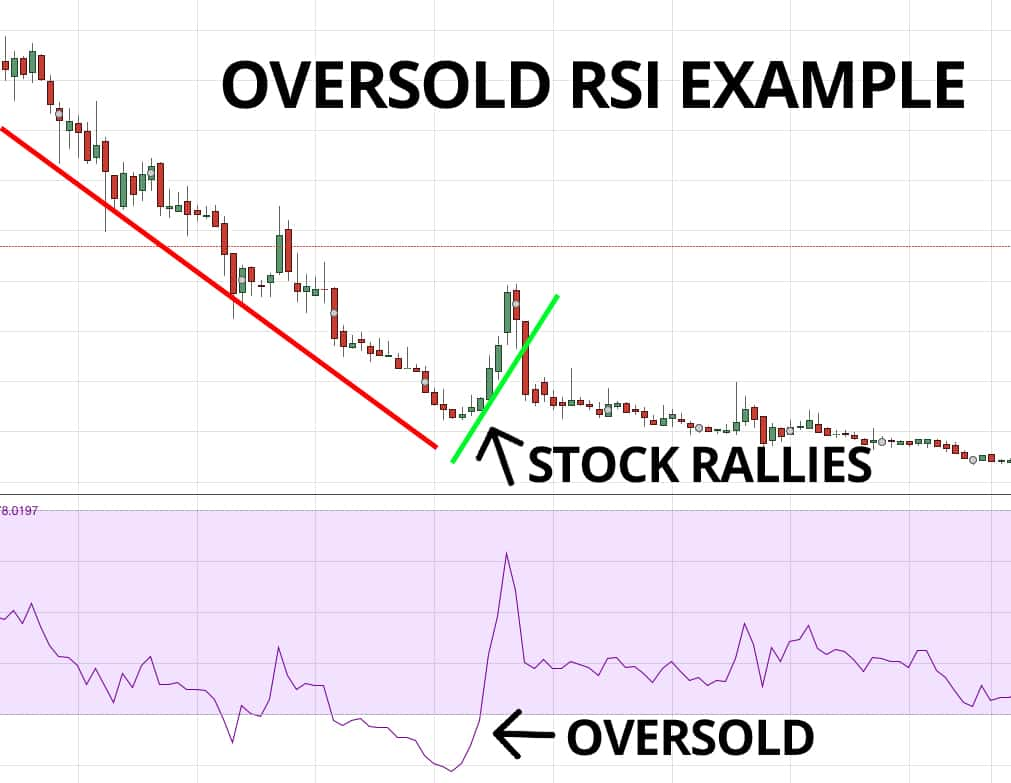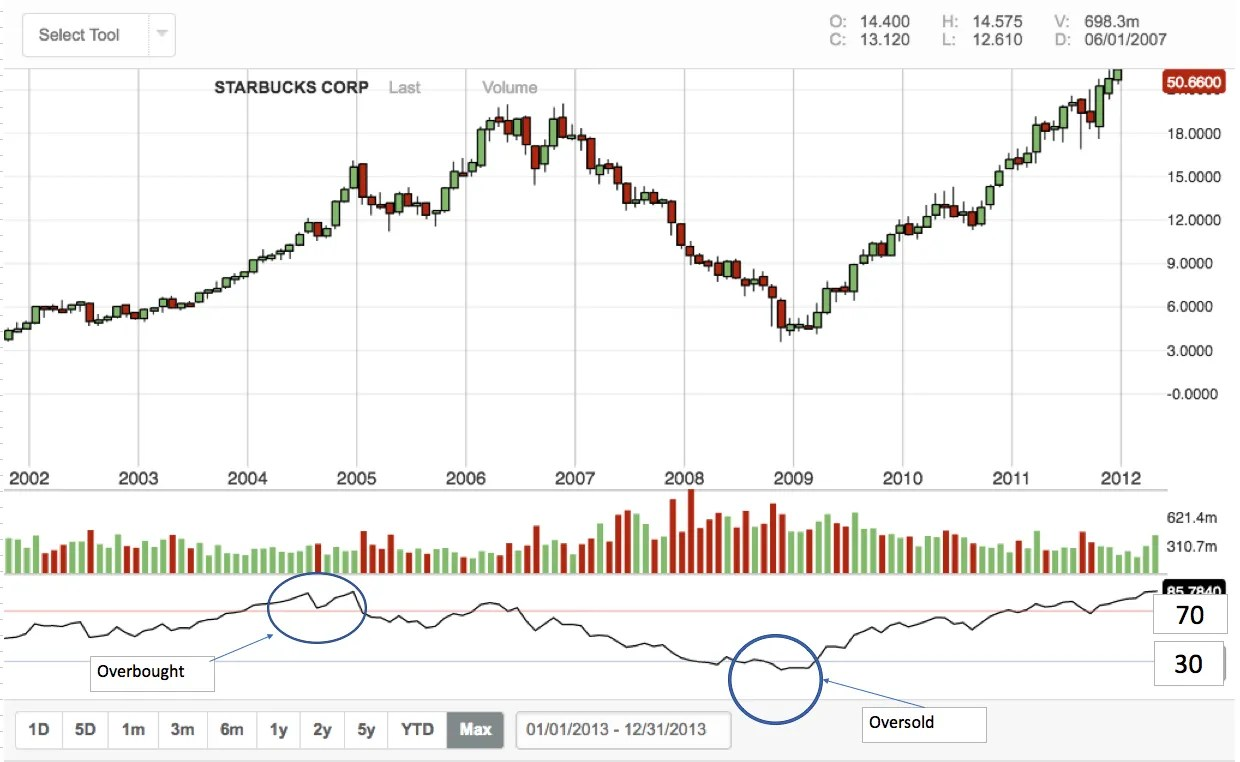# What is the Relative Strength Index?

If you are just getting into the world of trading, whether it is forex cryptocurrencies, the stock market or anything in between, one of the most important things that you need to be familiar with are the different indicators out there. Indicators are of course very useful tools that can tell you all about the volatility, volume, momentum or trend of a specific asset or security. Now, one of the most useful indicators that you should know how to use is the relative strength index indicator.

Now, when it comes to indicators that are very user friendly and reliable, the relative strength index indicator is indeed one of the best. However, with that being said, you still need to know exactly what that relative strength index indicator tells you how to read it, how to calculate it and more.

There are also some big mistakes that many people make when using this relative strength index indicator, and we are going to tell you how to avoid these mistakes. Right now we want to provide you with a good basic foundation of what the RSI or relative strength index indicator is all about. This is your introduction to the RSI indicator.## What is the Relative Strength Index Indicator?

Welles Wilder was the man developed this indicator and introduced it to the trading community in his 1978 book, “New Concepts in Technical Trading Systems”. The relative strength index indicator is one of the most important momentum indicators out there.

So right off the bat, what you know about the RSI is that it is a momentum indicator. Or in other words it tells you about the strength or the speed of price movement. To put it in simplest terms, it can tell you exactly how strong a specific trend is.

What you also need to know here is that the relative strength index indicator is often called an oscillator. The reason is because the value oscillates between 0 and 100.

For this reason, the RSI is somewhat similar to the Stochastic indicator. The RSI indicator is a type of indicator that will tell you whether a security or asset is overbought or oversold. If you’re looking at an RSI indicator chart, the higher that relative strength index value is, the faster a price is going up. The lower that RSI value, the faster the price is going down.## Calculating the RSI

Of course, one of the most important things that you need to know here is how to calculate the relative strength index. We have listed the formula for at this indicator below.

100 – 100/[1 + RS]

The one thing that you need to remember here is that RS stands for the average gain divided by the average loss. This might seem like a bit of a confusing formula, especially the final part of the equation, but with that being said, with a little bit of practice you should be able to master it without much issue.## How the Relative Strength Index Indicator Works

OK, so we have already established that this type of indicator is a momentum indicator that measures the number of recent price changes in terms of the average gains divided by the average losses over a given period of time.

What is also important to know is that this is known as a leading indicator, which means that the signals usually come after a price movement, not before, thus providing you with early signals for coming trades, but the drawback is that there are often false signals or premature signals. Due to this drawback, it is very important that the relative strength indicator be used with other technical analysis indicators and tools in order to confirm your findings.

Now, in terms of the value of the relative strength index indicator, what you need to know here is that if the value is above 70, it generally means that the security is becoming overbought or overvalued . This could be due to a corrective pullback or a trend reversal. Now many traders also see this as an indication that a trend on the rise is likely to reverse in the near future, and therefore it could be a good time to sell.On the other hand, if the value of the relative strength index indicator is below 30, then it indicates that an asset or security is either undervalued or oversold. This therefore increases the likelihood of the price going up in the near future, and traders will often interpret this as a sign of that a falling trend is going to reverse. Therefore, providing you with an opportunity to place a goodbye trade.

When using the RSI indicator, the other thing that you need to look out for are the middle crossovers, or in other words, movements around the center line, which is the 50 line. For instance, a rising centerline crossover comes upwards when the RSI value crosses above 50. Now as the value continues to rise toward 70, it means that the market trend is growing in strength and this is seen as a bullish signal until the RSI gets to the 70 line. Remember, the higher the RSI value is, the stronger a trend is.

So if we are talking about movement that happens from above the centerline and goes down towards the 30 line, it means that a market trend is losing momentum, which can therefore be interpreted as a bearish signal, until the value goes down to 30.## A Great Way to Use The RSI

Right now we want to provide you with some great tips for using the relative strength index indicator so you can start making money with it. Just remember here is that when the value of the RSI is over 50, it usually always indicates that the average gains are higher than the average losses. So how do you use this indicator to win trades? Well, personally we recommend using the relative strength index indicator as a trend filter.

To do this you should turn the period of two 200. Such a long period will allow you to identify the average gains to average losses over a very long period of time. Now when using this 200. If you see that the value of the relative strength index indicator is over 50, then you can assume that the market is currently in an upward trend and the higher that value is, the stronger the trend. If the value is below 50, then you can assume that the market is trending downwards.

## Final Thoughts on The Relative Strength Index Indicator

Quite admittedly, we have only provided you with a very basic framework of what this relative strength index indicator is all about. But with that being said, there is a whole lot to know here, and we could probably write a whole book on the matter. The bottom line here is that if you want to become a profitable trader, then you absolutely need to know how to use this RSI indicator.

If you need help day trading, and what you need is a comprehensive education, particularly on Forex trading, then the best place to be is the Income Mentor Box Day Trading Academy. At this time, the IMB Academy is the most comprehensive, user friendly, effective, and affordable Forex trading school out there.

### CLICK BELOW TO JOIN INCOME MENTOR BOX AND START MAKING REAL PROFITS!